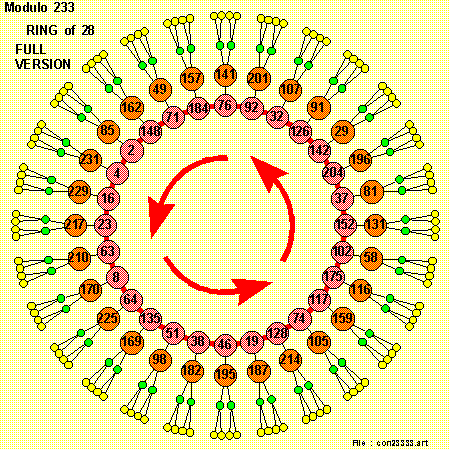# An Approach to the Characteristics of Numbers

## Finding #3 : Fermat Numbers

• ### We then have the factorization of the 5th , 6th & 7th Fermat Numbers :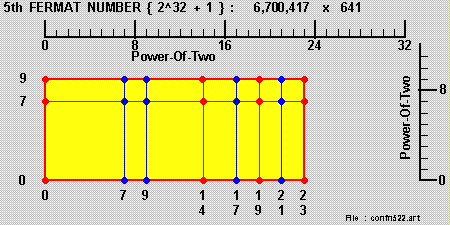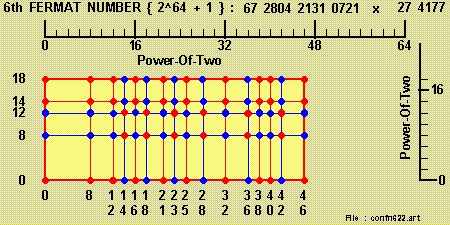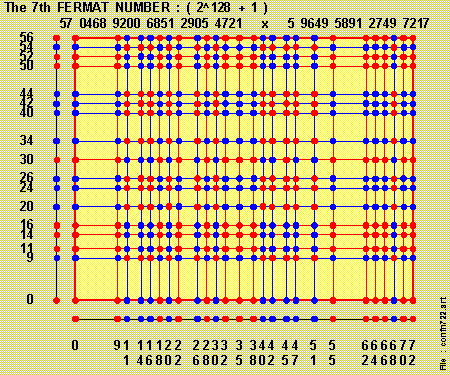## Finding #4 - The Fermat Little Theorem

### We first place 12 [ 1's ] , 12 [ 2's ] , ...... , 12 [ 12's ] on the diagonals of 12 [ 12 x 12 ] 'Square Boxes'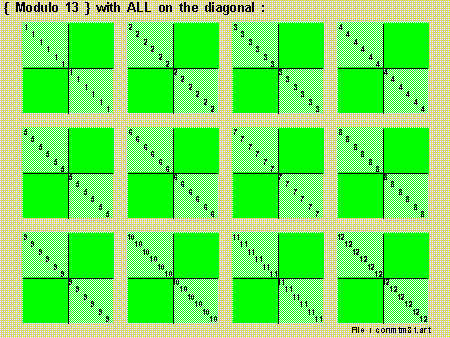### We then 'swung-out' the numbers from the 'diagonals' to the appropriate places: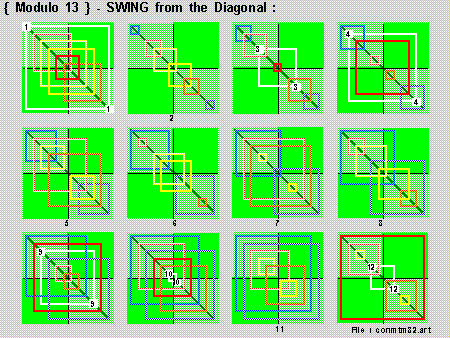## Finding #5 - Squares-Of-Squares

• ### for the 'prime' number { 233 = 8 * 29 + 1 } the equation :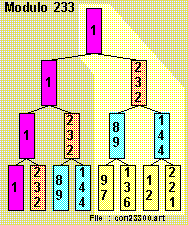### This latter group is then known as the 'RING-OF-28' :